Are you looking for the best Maths NCERT Solutions Chapter 3 Ex 3.2 Class 10? Then, grab them from our page and ace up your preparation for CBSE Class 10 Exams.

These Solutions are part of RS Aggarwal Solutions Class 10. Here we have given RS Aggarwal Solutions Class 10 Chapter 3 Linear equations in two variables Ex 3B. You must go through NCERT Solutions for Class 10 Maths to get better score in CBSE Board exams along with RS Aggarwal Class 10 Solutions.

## RS Aggarwal Class 10 Solutions Chapter 3 Linear equations in two variables Ex 3B

Solve for x and y:
Question 1.
Solution:
x + y = 3 …..(i)
4x – 3y = 26 …(ii)
From (i), x = 3 – y
Substituting the value of x in (ii),
4(3 – y) – 3y = 26
=> 12 – 4y – 3y = 26
-7y = 26 – 12 = 14
y = -2
x = 3 – y = 3 – (-2) = 3 + 2 = 5
Hence, x = 5, y = -2

Question 2.
Solution:Question 3.
Solution:
2x + 3y= 0 ……..(i)
3x + 4y = 5 …….(ii)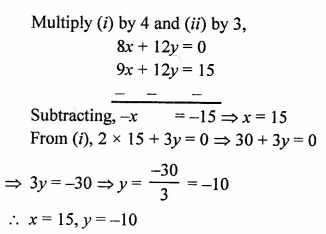Question 4.
Solution:
2x – 3y = 13 ……(i)
7x – 2y = 20 ……….(ii)Question 5.
Solution: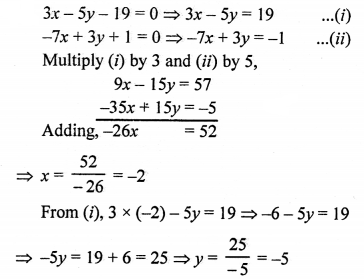=> x = -2, y = -5

Question 6.
Solution: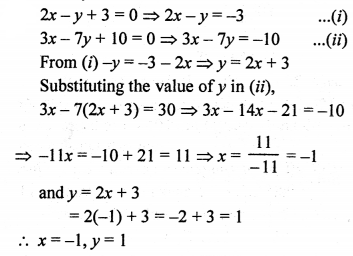Question 7.
Solution: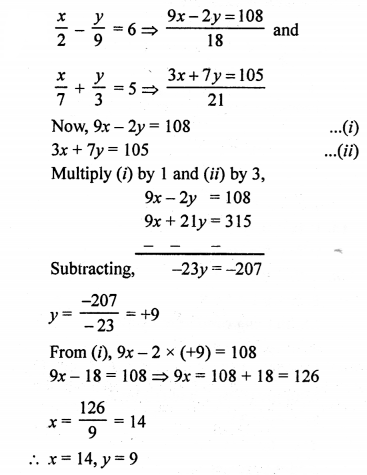Question 8.
Solution: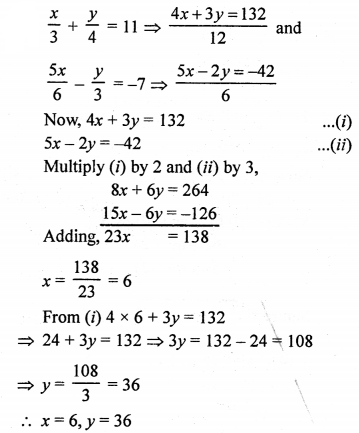Question 9.
Solution: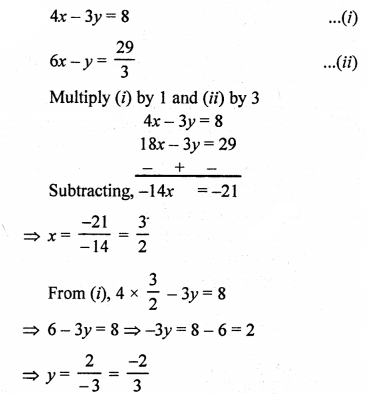Hence, x = $$\frac { 3 }{ 2 }$$ , y = $$\frac { -2 }{ 3 }$$

Question 10.
Solution: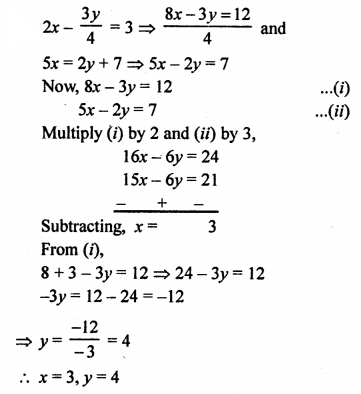Question 11.
Solution: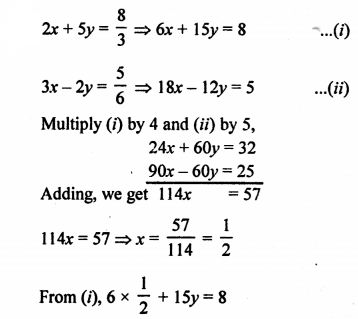Question 12.
Solution: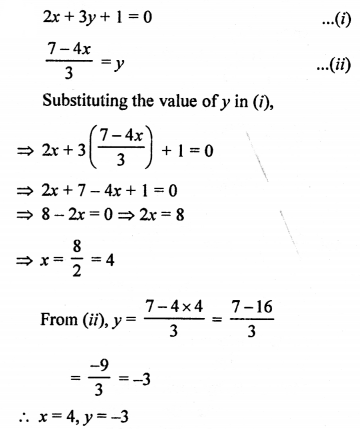Question 13.
Solution:
0.4x + 0.3y = 1.7
0.7x – 0.2y = 0.8
Multiplying each term by 10
4x + 3y = 17
0.7x – 2y = 8
Multiply (i) by 2 and (ii) by 3,
8x + 6y = 34
21x – 6y = 24
29x = 58
x = 2
From (i) 4 x 2 + 3y = 17
=> 8 + 3y = 17
=> 3y = 17 – 8 = 9
y = 3
x = 2, y = 3

Question 14.
Solution:Question 15.
Solution: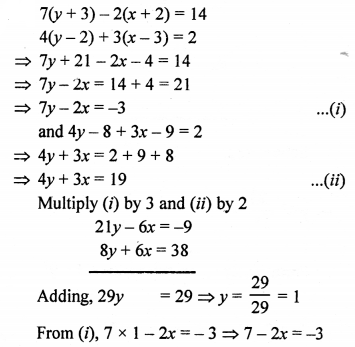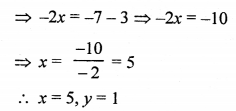Question 16.
Solution:
6x + 5y = 7x + 3y + 1 = 2(x + 6y – 1)
6x + 5y = 7x + 3y + 1
=> 6x + 5y – 7x – 3y = 1
=> -x + 2y = 1
=> 2y – x = 1 …(i)
7x + 3y + 1 = 2(x + 6y – 1)
7x + 3y + 1 = 2x + 12y – 2
=> 7x + 3y – 2x – 12y = -2 – 1
=> 5x – 9y = -3 …..(ii)
From (i), x = 2y – 1
Substituting the value of x in (ii),
5(2y – 1) – 9y = -3
=> 10y – 5 – 9y = -3
=> y = -3 + 5
=> y = +2
x = 2y – 1 = 2 x 2 – 1 = 4 – 1 = 3
x = 3, y = 2

Question 17.
Solution:and x = y – 4 = 6 – 4 = 2
x = 2, y = 6

Question 18.
Solution: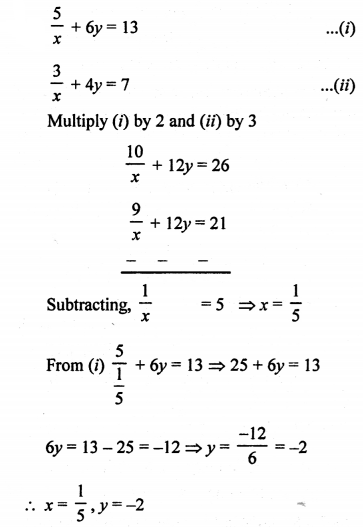Question 19.
Solution: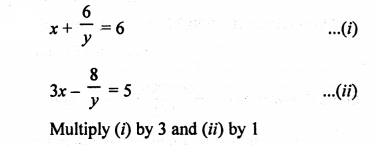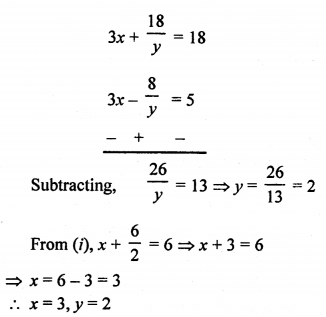Question 20.
Solution: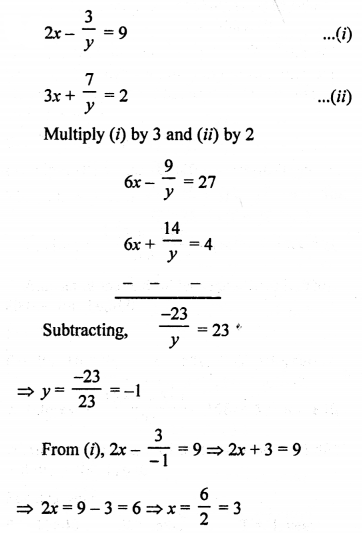x = 3, y = -1

Question 21.
Solution: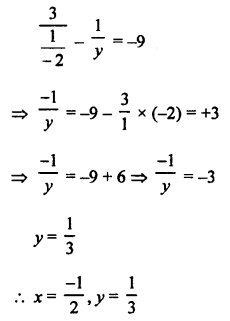Question 22.
Solution: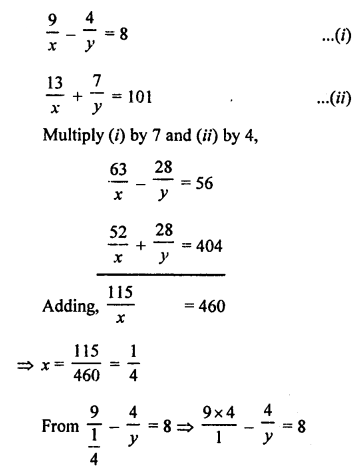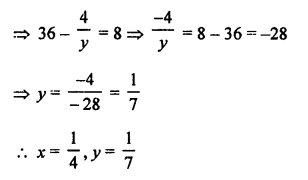Question 23.
Solution: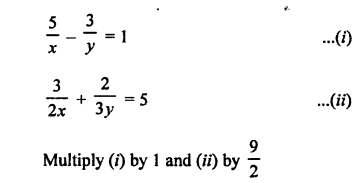Question 24.
Solution: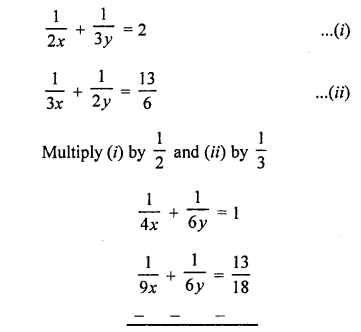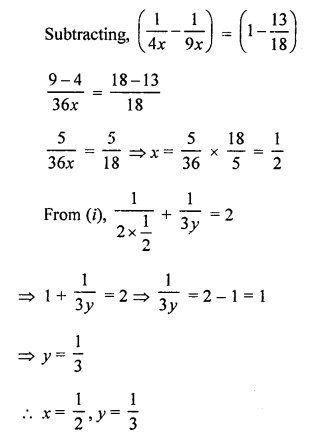Question 25.
Solution:Question 26.
Solution: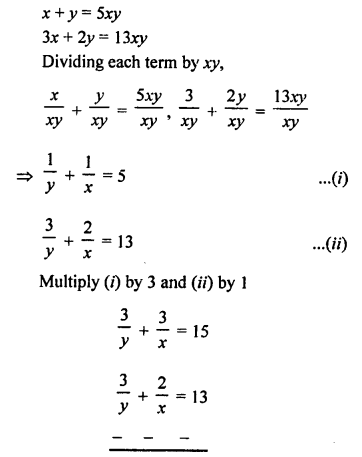Question 27.
Solution: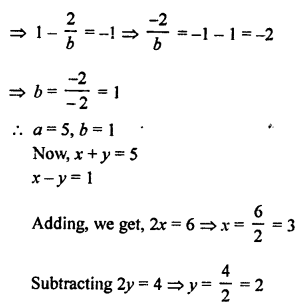x = 3, y = 2

Question 28.
Solution: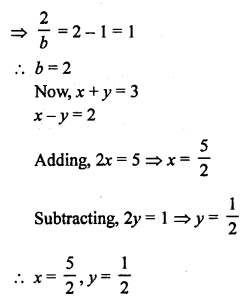Question 29.
Solution:Question 30.
Solution: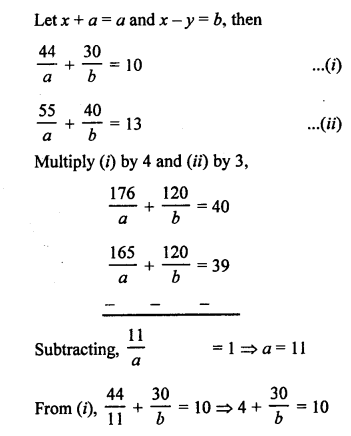Question 31.
Solution: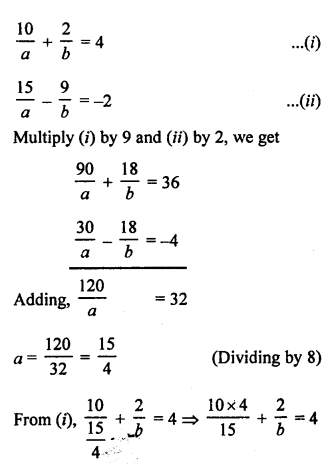Question 32.
Solution:
71x + 37y = 253
37x + 71y = 287
108x + 108y = 540
x + y = 5 ………(i) (Dividing by 108)
and subtracting,
34x – 34y = -34
x – y = -1 ……..(ii) (Dividing by 34)
2x = 4 => x = 2
and subtracting,
2y = 6 => y = 3
Hence, x = 2, y = 3

Question 33.
Solution:
217x + 131y = 913 …(i)
131x + 217y = 827 …..(ii)
348x + 348y = 1740
x + 7 = 5 …..(iii) (Dividing by 348)
and subtracting,
86x – 86y = 86
x – y = 1 …(iv) (Dividing by 86)
2x = 6 => x = 3
and subtracting,
2y = 4 => y = 2
x = 3, y = 2

Question 34.
Solution:
23x – 29y = 98 ……(i)
29x – 23y = 110 ……(ii)
52x – 52y = 208
x – y = 4 ……(iii) (Dividing by 52)
and subtracting
-6x – 6y = -12
x + y = 2 …..(iv)
2x = 6 => x = 3
Subtracting (iii) from (iv)
2y = -2 => y = -1
x = 3, y = -1

Question 35.
Solution: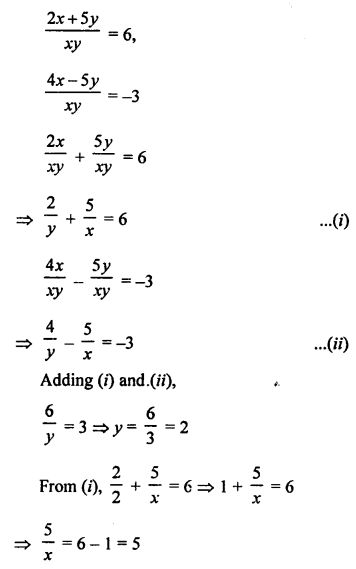x = 1 and y = 2

Question 36.
Solution:Question 37.
Solution: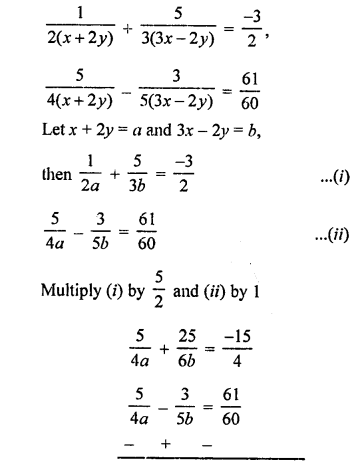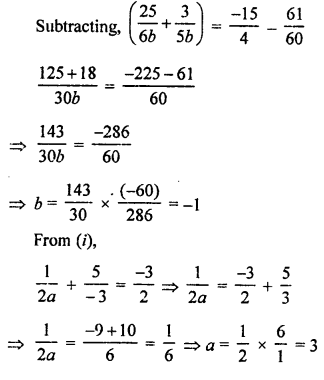Question 38.
Solution:Question 39.
Solution: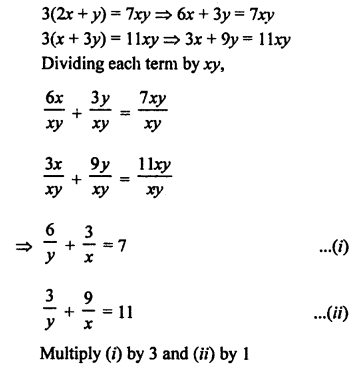Question 40.
Solution:Question 41.
Solution: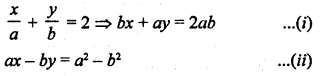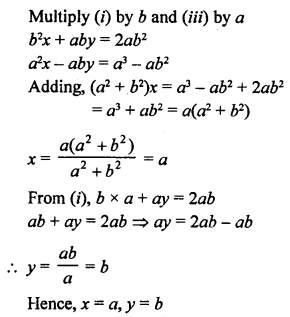Question 42.
Solution: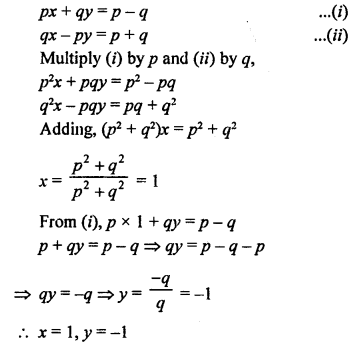Question 43.
Solution: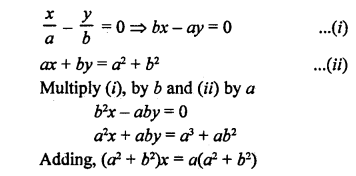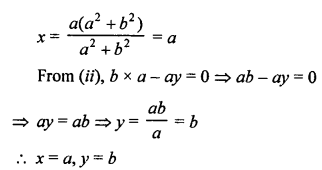Question 44.
Solution: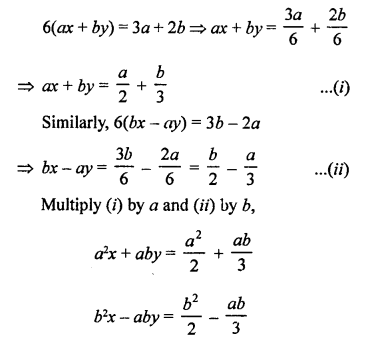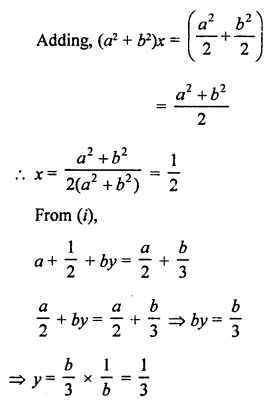a = $$\frac { 1 }{ 2 }$$ , b = $$\frac { 1 }{ 3 }$$

Question 45.
Solution: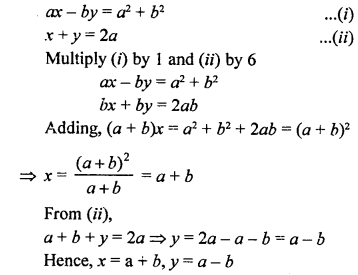Question 46.
Solution: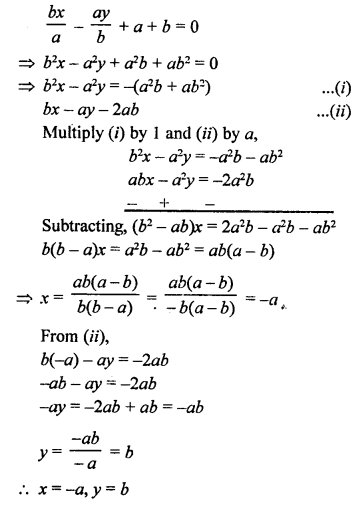Question 47.
Solution: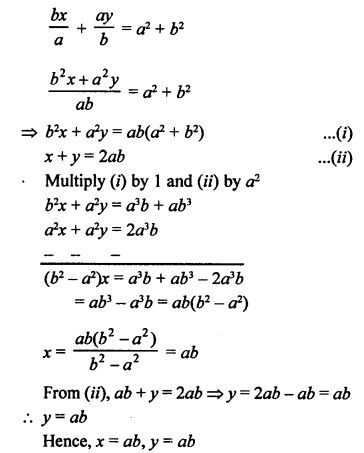Question 48.
Solution: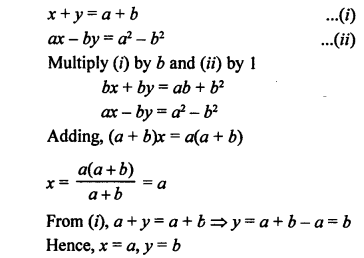Question 49.
Solution:
a²x + b²y = c² ……(i)
b²x + a²y = d² …….(ii)
Multiply (i) by a² and (ii) by b²,Question 50.
Solution: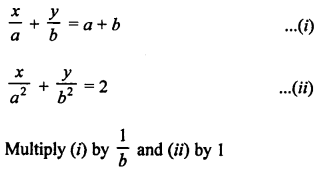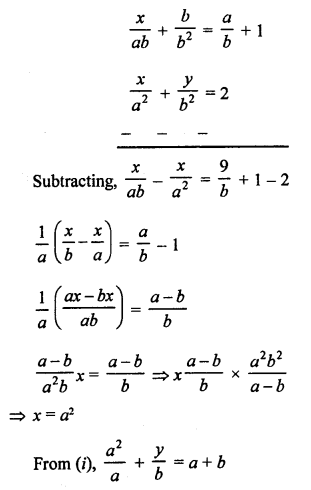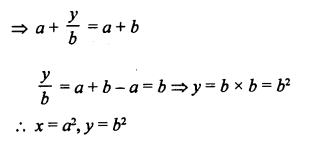Hope given RS Aggarwal Solutions Class 10 Chapter 3 Linear equations in two variables Ex 3B are helpful to complete your math homework.

If you have any doubts, please comment below. Learn Insta try to provide online math tutoring for you.